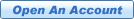Volatility

Volatility can be a very important factor in deciding what kind of options to buy or sell. Volatility shows the investor the range that a stocks price has fluctuated in a certain period. The official mathematical value of volatility is denoted as "the annualized standard deviation of a stocks daily price changes."

There are two types of Volatility: Statistical Volatility and Implied Volatility.

Statistical Volatility - a measure of actual asset price changes over a specific period of time.

Implied Volatility - a measure of how much the "market place" expects asset price to move, for an option price. That is, the volatility that the market itself is implying.

The computation of volatility is a difficult problem for mathematical application.

In the Black-Scholes model, volatility is defined as the annual standard deviation of the stock price. There is a way in which the strategist can let the market compute the volatility for him. This is called using the implied volatility - that is, the volatility that the market itself is implying. This is similar to an efficient market hypothesis. If there is enough trading interest in an option that is close to the money, that option will generally be fairly priced.

The Black-Scholes Formula

The Black-Scholes formula was the first widely-used model for option pricing. This formula can be used to calculate a theoretical value for an option using current stock prices, expected dividends, the option's strike price, expected interest rates, time to expiration and expected stock volatility. While the Black-Scholes model does not perfectly describe real-world options markets, it is still often used in the valuation and trading of options.

The variables of the Black Scholes formula are:

• Stock Price
• Strike Price
• Time remaining until expiration expressed as a percent of a year
• Current risk-free interest rate
• Volatility measured by annual standard deviation.

The Greeks

The Greeks are a collection of statistical values (expressed as percentages) that give the investor a better overall view of how a stock has been performing. These statistical values can be helpful in deciding what options strategies are best to use. The investor should remember that statistics show trends based on past performance. It is not guaranteed that the future performance of the stock will behave according to the historical numbers. These trends can change drastically based on new stock performance.

Beta: a measure of how closely the movement of an individual stock tracks the movement of the entire stock market.

Delta: The Delta is a measure of the relationship between an option price and the underlying stock price. For a call option, a Delta of .50 means a half-point rise in premium for every dollar that the stock goes up. For a put option contract, the premium rises as stock prices fall. As options near expiration, in the money contracts approach a Delta of 1.

In this example the delta for stock XYZ is 0.50. As the price of the stock changes by \$2.00 the price of the options will change by 50 cents for every dollar. Therefore the price of the options will change by (.50 x 2) = 1.00. The call options will have their price increased by \$1.00 and the put options will have their price decreased by \$1.00. The Delta is not a fixed percentage. Changes in price of stock and time to expiration will have an effect on the delta value.

Gamma: Sensitivity of Delta to unit change in the underlying. Gamma indicates an absolute change in delta. For example, a Gamma change of 0.150 indicates the delta will increase by 0.150 if the underlying price increases or decreases by 1.0. Results may not be exact due to rounding.

Lambda: A measure of leverage. The expected percent change in the value of an option for a 1 percent change in the value of the underlying product.

Rho: Sensitivity of option value to change in interest rate. Rho indicates the absolute change in option value for a one percent change in the interest rate. For example, a Rho of .060 indicates the option's theoretical value will increase by .060 if the interest rate is decreased by 1.0. Results may not be exact due to rounding.

Theta: Sensitivity of option value to change in time. Theta indicates an absolute change in the option value for a 'one unit' reduction in time to expiration. The Option Calculator assumes 'one unit' of time is 7 days. For example, a theta of -250 indicates the option's theoretical value will change by -.250 if the days to expiration is reduced by 7. Results may not be exact due to rounding. NOTE: 7 day Theta changes to 1 day Theta if days to expiration is 7 or less.

Vega (kappa, omega, tau): Sensitivity of option value to change in volatility. Vega indicates an absolute change in option value for a one percent change in volatility. For example, a Vega of .090 indicates an absolute change in the option's theoretical value will increase by .090 if the volatility percentage is increased by 1.0 or decreased by .090 if the volatility percentage is decreased by 1.0. Results may not be exact due to rounding.

Important Note: Options involve risk and are not suitable for all investors. For more information, please read the Characteristics and Risks of Standardized Options